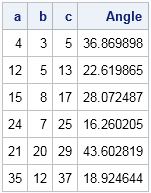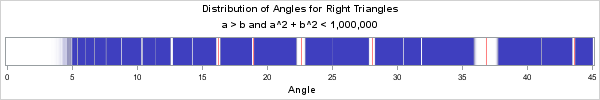Last week I was chatting with some mathematicians and I mentioned the blog post that I wrote last year on the distribution of Pythagorean triples.

In my previous article, I showed that there is an algorithm that uses matrix multiplication to generate every primitive Pythagorean triple by starting with the simple (3,4,5) right triangle. The algorithm does not systematically generate triangles with large legs from triangles with smaller legs. Instead, it might generate several triangles with large legs, followed by one or more triangles with small legs. Given a specific radius r, it would be interesting to prove how many iterations of the algorithm are required to guarantee that the algorithm has generated all primitive Pythagorean triples whose hypothesis is less than r.

From a statistical point of view, it is interesting to estimate the distribution of the smallest angle in primitive Pythagorean right triangles. Suppose that you consider only the primitive triangles whose hypotenuse is less than 106. The following histogram shows the distribution of the smallest angle (arctan(b/a) if a is the longer leg) for angles ranging from 0 to 45 degrees in increments of 0.5 degrees. The histogram is formed from 98,151 triangles, which is considerably less than the 159,139 primitive right triangles whose hypotenuses are less than one million.The histogram has several interesting features:

• Apparently there are relatively few long-and-skinny triangles. In this sample, few have angles less than 5 degrees. The smallest angle is 3.47 degrees, which corresponds to the (33, 544, 545) right triangle.
• The distribution is not uniform. In fact, there are noticeable gaps in the density distribution. The biggest gaps are near 37, 23, and 28 degrees.
• Some Pythagorean triangles that are nearly isosceles. In this sample, the (137903, 137904, 195025) triangle has an angle equal to 44.99979 degrees. This triangle is an example of a twin-leg Pythagorean triple in which the legs lengths differ by 1.

The gaps are very interesting. I conjecture that the gaps in the distribution are not random, but that they are related to low-order triangles. The following statements compute the smallest angle of some right triangles whose legs are small integers:

```proc iml; v = { 4 3 5, 12 5 13, 15 8 17, 24 7 25, 21 20 29, 35 12 37 }; a = atan2(v[,2], v[,1]) * 180/constant('pi'); print v[L="" c={"a" "b" "c"}] a[L="Angle"];```If you overlay lines at each of the angles above, they fall right into the gaps of the histogram. The (3,4,5) triangle is centered at the biggest gap (37 degrees). The (5, 12, 13) triangle is responsible for the next largest gap (23 degrees), and so forth. You can overlay these lines on the histogram, or overlay the lines on a fringe plot, as shown in the following image in which the red lines represent the angles of six low-order triangles:If this histogram is representative of the true density distribution of the smallest angle in a Pythagorean triangle, the low-order triangles seem to be surrounded by a region of reduced density. If I were to study this problem further, I would conjecture that the gap induced by a low-order triangle is related to Farey sequences. Farey sequences arise in the darndest places, ranging from the dynamics of planetary objects to the study of Diophantine equations. In fact, the fringe plot reminds me of the gaps and divisions in Saturn's rings, which is another place where Farey sequences have an application. Of course, these gaps could also be an artifact of the algorithm that I am using to generate the primitive Pythagorean triangles, but that can be checked by using a different algorithm to generate the Pythagorean triples. Personally, I think the gaps are produced by a real number-theoretic phenomenon.

The density distribution of the smallest angle is interesting, don't you think? Mathematicians who work in number theory can probably explain why the gaps exist, but they were a surprise to me. Who would have guessed that Pythagorean triangles would lead to such an interesting distribution? It's a beautiful connection between probability, statistics, and high-school geometry.

Addendum 16APR2015: Well, my conjecture was wrong. Ian Wakeling implemented a different algorithm for generating all primitive Pythagorean triples that have a hypotenuse length that is less than 1,000,000. I have linked to his SAS/IML program. His program indicates that the angles of the Pythagorean triangles are uniformly distributed in the interval (0, 45) degrees, which is itself an interesting result! The artifacts that I saw are a result of the algorithm that I was using to construct the triples, not a property of the triples themselves. Thanks, Ian!

The algorithm that Ian used enables you to investigate "twin-leg triangles" in which the legs differ by 1. For long legs, these triangles are nearly isosceles. Or investigate "twin leg-hypotenuse triangles" in which the hypotenuse and the longer leg differ by 1. For long legs, these triangles are long and skinny. It is also interesting to ask questions such as "which number is associated with a lot of Pythagorean triangles" (60,060 is one).

ShareDistinguished Researcher in Computational Statistics

Rick Wicklin, PhD, is a distinguished researcher in computational statistics at SAS and is a principal developer of PROC IML and SAS/IML Studio. His areas of expertise include computational statistics, simulation, statistical graphics, and modern methods in statistical data analysis. Rick is author of the books Statistical Programming with SAS/IML Software and Simulating Data with SAS.

1.Ian Wakeling on

Thank you for posting such an interesting article. Here is a method of calculating all 159,139 triples at once using 1000x1000 matrices and a lot of memory. the method is based on Theorem 11.1 page 394 from Kenneth Rosen's 1984 book "Elementary Number Theory and its Applications".
.
a = 1000; /* sqrt of maximum hypotenuse */
m = repeat(t(1:a), 1, a);
n = repeat(1:a, a);
good = loc( (gcd(m, n)=1) # mod(m - n, 2) # (m > n) # ((m#m + n#n)<=(a#a)) );
m = m[good];
n = n[good];
t = (2#m#n) || (m#m - n#n) || (m#m + n#n);
create triples from t;
append from t;
.
If I use this larger set of triples to make a distribution of angles, then the profile is virtually uniform between zero and 45 degrees, so I am thinking that there must be something specific to your algorithm that makes it avoid triangles that are similar in shape to the lower-order ones.

•That is so awesome! Thanks for investigating this problem so quickly.

2.Rick, I was curious about the question you posed at the beginning of the article. I think the general problem is way too hard for me, but I can try to make some progress by working on r=1E6. The algorithm can be reversed by using the inverse of L1, L2 & L3, and it is possible to map out, for every triple, the length of the path going back up the tree to (3, 4, 5). Doing this for all 159,169 triples, I can show the depth vs the cumulative percentage of triples the algorithm finds:
.
Depth %
15 61.68
20 76.51
30 87.65
40 92.03
60 95.60
80 97.04
120 98.35
200 99.22
300 99.60
500 99.87
705 100.00
.
I found it surprising that such a large depth is necessary, and in fact the final level returns just a single triple, the one with the smallest angle. You can verify this with the code:
.
x = {3 4 5} * ( L1**705 );
.
which gives the triple (1413, 998284, 998285). Not often that you get to take the 705th power of a matrix!

3.It’s very interesting your article, I was looking for Pythagorean triple triangle with an entire angle, and I can’t find yet the way to solve it I thing may be is the same solution for degrees or radian angles. I will be grateful if to share to me some idea if it exist

4.Goldtree St Bach on

if c is prime, there will be only one pythagorean triplet, if it is composite, more than one.

thanks

5.Nice article, Rick. I'm curious about the angle difference of PTs and/or PPTs (Pythagorean triples and primitive Pythagorean triples, resp.).
In particular, do any PTs differ by pi/4?
Any ideas how to go about finding if any exist and if so, which ones?

Thanks!